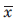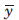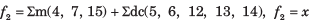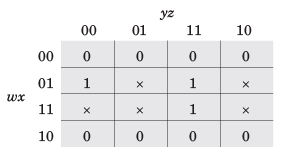A switching function of four variable, f (w, x y,
z) is to equal the product of two other function f1and f2, of the same variable f = f1f2.
The function f and f1are as follows :
f =∑m(4,7,15) ,
f1 =∑m(0,1,2, 3, 4,7, 8,9,10,11,15)
Que: The simplest function for f2 is
a)  x
b)c)  y
d)### Related TestMachine Experts Feb 18, 2022Anusri Kothuri As Sep 21, 2018
From the simplification , f2=£m(4,7,15)+d(5,6,12,13,14) from the minimization of f2 we get f2=x